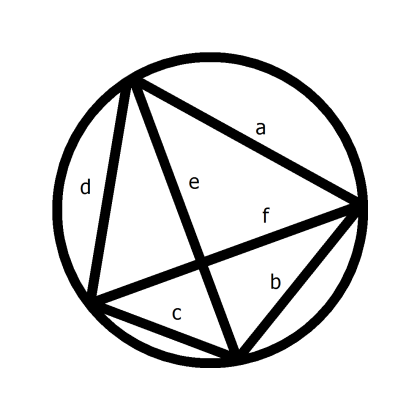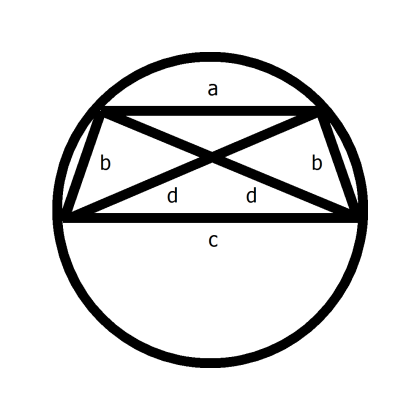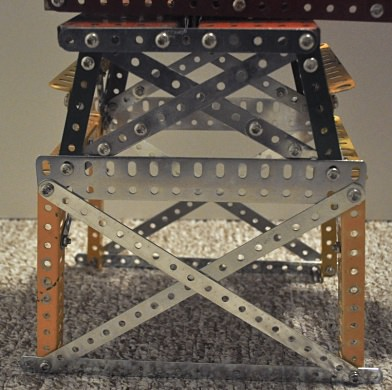# A Little More Mathematics for Meccano

Not a lot more, I promise.

In my earlier article I referred to Pythagoras’ theorem, and how it could be used to create right-angled triangles from standard Meccano strips or girders.

It turns out there is another rule, Ptolemy’s Theorem, which is just as useful. I’d never heard of it until I saw it in a Numberphile video on YouTube (a series I heartily recommend, by the way). It certainly wasn’t taught to me at school. This is probably because it is much trickier to prove than it is to state, so I’ll just state it.

Ptolemy’s theorem applies to quadrilaterals — shapes with four sides — that have all four corners lying on the same circle. In this case, the product of the quadrilateral’s two diagonals equals the sum of the products of the pairs of opposite sides. Sounds a bit like Pythagoras, doesn’t it?Figure 1 General quadrilateral on a circle

So, looking at Figure 1:

a × c + b × d = e × f

In fact, if the quadrilateral is a rectangle, because of the rectangle’s symmetry, it can be shown that Ptolemy reduces to Pythagoras. In other words, Pythagoras is a special case of Ptolemy, which is therefore the wider theorem. Move over, Pythagoras!

Where it becomes useful to us is in building a tapering tower with cross-bracing, with every piece an exact multiple of half-an-inch. (The usual rule applies of lengths being measured between end-hole centres as opposed to overall lengths, and, as just noted, in half-inch multiples).Figure 2 Quadrilateral with two parallel and two equal sides

Referring to Figure 2, our tapering section is a quadrilateral with two parallel unequal sides, being the top and bottom, the other two sides being the same length.

By symmetry, this shape will sit with all four corners on a circle, so Ptolemy applies. I’ve drawn the quadrilateral as being wider than it is tall, but it doesn’t have to be.

Because the diagonals are also the same length as each other:

a × c + b × b = d × d

Or, applying a bit of school algebra:

a × c = d² – b² = (d – b) × (d + b)

So you could simply make a = d – b and c = d + b

However, this will simply give you a flat shape with all four corners on the same straight line, on a circle of infinite radius. So you need d – b and/or d + b not to be prime numbers so you can swap factors from one to the other, to give you a more convenient shape.

Examples are 7 (top), 8 (bottom), 5 (sides) and 9 (diagonals), and 9, 12, 6 and 12. The first is based on d = 9 and b = 5 with a factor of 2 swapped between a and c. The second is d = 4 and b = 2, again with a factor of 2 swapped across, and the whole thing tripled. Indeed, you can double, triple, and so on, any of the sets of numbers and it will still work. See the close-up photograph of one of my models below for these examples in practice.Ptolemy’s Theorem in action in a model

Beyond this, other examples can be found ‘by inspection’, as my maths master used to say with unshakeable optimism.

A slight drawback is a that lengths don’t always work out to match standard strips, so you need to build up from smaller ones (or trim longer ones with a hacksaw, not that you would of course). Also, I can’t find an example where the diagonals are split into two integer halves, so you can drop in a bolt exactly at the crossing point, and it may be that it never happens. Is there a proof either way? An exercise for the reader perhaps.

On the plus side (no pun intended), there may be some near-miss combinations, where the perforations line up closely enough for the bolts to slip in (like 15, 26, 30 for a right-angled triangle). I don’t know; I haven’t looked yet, but I’d expect them to be quite large.

In all, a useful bit of maths. I’m just surprised I didn’t come across it sooner.

It is relatively straightforward to write a simple computer program to exhaustively search for integer solutions to Ptolemy’s theorem, that is, solutions for which all of c, a, b and d in Figure 2 of the original posting are whole numbers. If we restrict the solutions to those which can be constructed from 12.5” or shorter strips only (that is, c, a, b and d are all less than or equal to 24), then there 158 solutions. This excludes rectangles (that is, the cases when c = a), the trivial cases of all four vertices lying on a straight line, and is further limited to the case of the upper horizontal being shorter than the lower horizontal (that is, restricted to c greater than a).

Amongst these solutions there are in fact several where the diagonals cross at an integer distance from their ends, so that you can place a bolt exactly at the crossing. I’ve listed all 11 of these solutions in the table below, in which the quantity ‘s’ is the distance along a diagonal from one of the bottom corners to the crossing point. Recall that all the quantities refer to the distances between hole centres as multiples of the distance between adjacent holes. For example, the first solution corresponds to a trapezium with sides c, a and b made from strips with 6, 4 and 8 holes respectively, with the diagonal strip having 9 holes.

c a b d s

-----------------------------------

5 3 7 8 5

8 4 7 9 6

8 7 13 15 8

10 6 14 16 10

12 3 8 10 8

15 9 11 16 10

15 9 21 24 15

15 12 12 18 10

16 5 19 21 16

16 8 14 18 12

24 6 16 20 16

The crossing points in these solutions are truly at exact integer distances. For aficionados, I’ll try to explain how you can verify this. Referring again to Figure 2, those that recall their school geometry will note that the triangle formed by the ends of the lower horizontal side and the diagonal crossing point is similar to the one formed by the ends of the upper horizontal side and the diagonal crossing point. The ratios of corresponding sides of the two triangles are therefore all the same, the ratio being that of the horizontal sides, namely c to a. The lengths of the two diagonal sections, s and (d-s) are therefore in the same ratio:

s / (d — s) = c / a

which, with a little algebraic rearrangement, yields

s = (c × d) / (c + a)

You can verify that all of the solutions satisfy this equation, as well as Ptolemy’s theorem

a × c + b × b = d × d

which was quoted in the original posting.